## three capacitors are connected in series to an ac voltage source with amplitude 12.0 V and frequency 6.3 kHz. What are the peak voltages acr

Question

three capacitors are connected in series to an ac voltage source with amplitude 12.0 V and frequency 6.3 kHz. What are the peak voltages across each capacitor

in progress 0
4 months 2021-09-05T05:53:34+00:00 1 Answers 4 views 0

The peak voltages across each capacitor are 6.0 V, 4.0 V and 2.0 V.

Explanation:

Given that,

Amplitude = 12.0 V

Frequency = 6.3 kHz

Suppose, Three capacitors are 2.0 μF, 3.0 μF and 6.0 μF

We need to calculate the equivalent capacitor

Using formula of series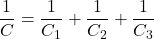Put the value into the formula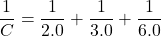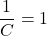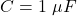We need to calculate the charge

Using formula of charge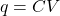Put the value into the formula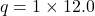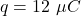The charge will be same in each capacitor because the capacitors are connected in series.

We need to calculate the voltage across first capacitor

Using formula of voltage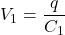Put the value into the formula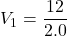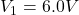We need to calculate the voltage across second capacitor

Using formula of voltage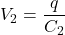Put the value into the formula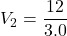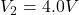We need to calculate the voltage across third capacitor

Using formula of voltage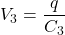Put the value into the formula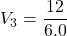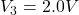Hence, The peak voltages across each capacitor are 6.0 V, 4.0 V and 2.0 V.